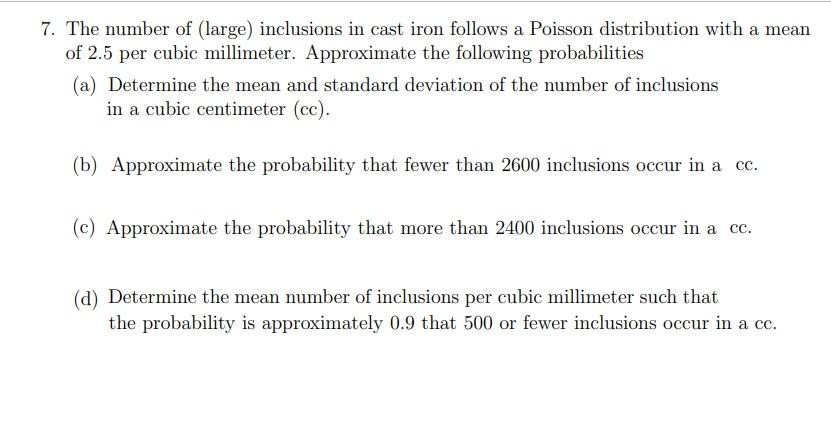# Question Solved1 Answer7. The number of large) inclusions in cast iron follows a Poisson distribution with a mean of 2.5 per cubic millimeter. Approximate the following probabilities (a) Determine the mean and standard deviation of the number of inclusions in a cubic centimeter (cc). (b) Approximate the probability that fewer than 2600 inclusions occur in a cc. (c) Approximate the probability that more than 2400 inclusions occur in a cc. (d) Determine the mean number of inclusions per cubic millimeter such that the probability is approximately 0.9 that 500 or fewer inclusions occur in a cc.GLX6V9 The Asker · Probability and StatisticsTranscribed Image Text: 7. The number of large) inclusions in cast iron follows a Poisson distribution with a mean of 2.5 per cubic millimeter. Approximate the following probabilities (a) Determine the mean and standard deviation of the number of inclusions in a cubic centimeter (cc). (b) Approximate the probability that fewer than 2600 inclusions occur in a cc. (c) Approximate the probability that more than 2400 inclusions occur in a cc. (d) Determine the mean number of inclusions per cubic millimeter such that the probability is approximately 0.9 that 500 or fewer inclusions occur in a cc.
More
Transcribed Image Text: 7. The number of large) inclusions in cast iron follows a Poisson distribution with a mean of 2.5 per cubic millimeter. Approximate the following probabilities (a) Determine the mean and standard deviation of the number of inclusions in a cubic centimeter (cc). (b) Approximate the probability that fewer than 2600 inclusions occur in a cc. (c) Approximate the probability that more than 2400 inclusions occur in a cc. (d) Determine the mean number of inclusions per cubic millimeter such that the probability is approximately 0.9 that 500 or fewer inclusions occur in a cc.